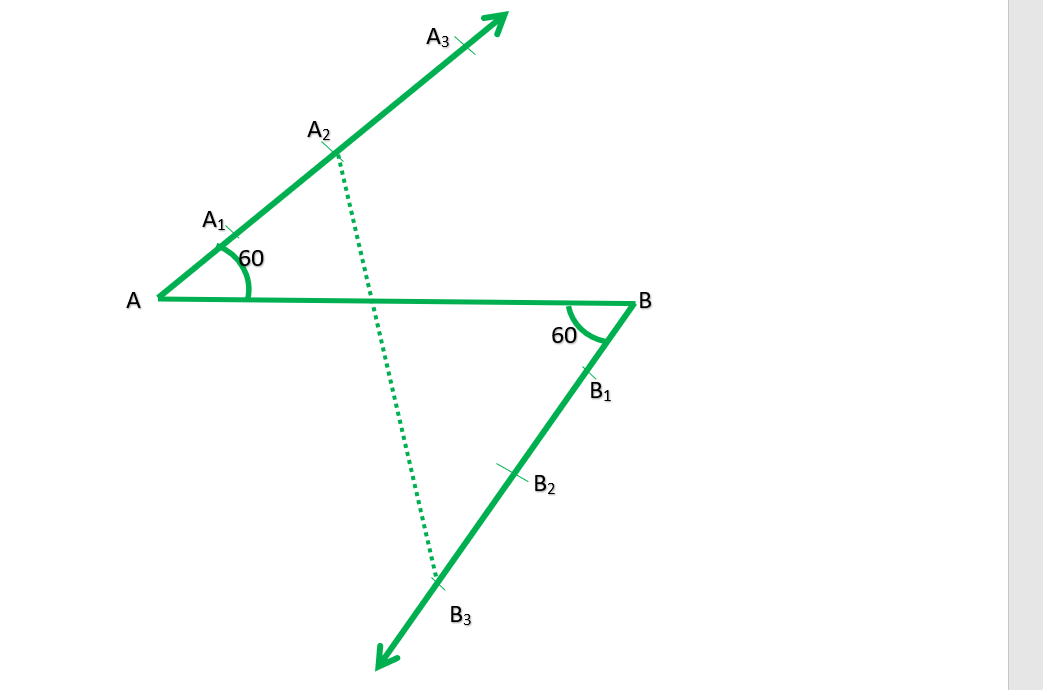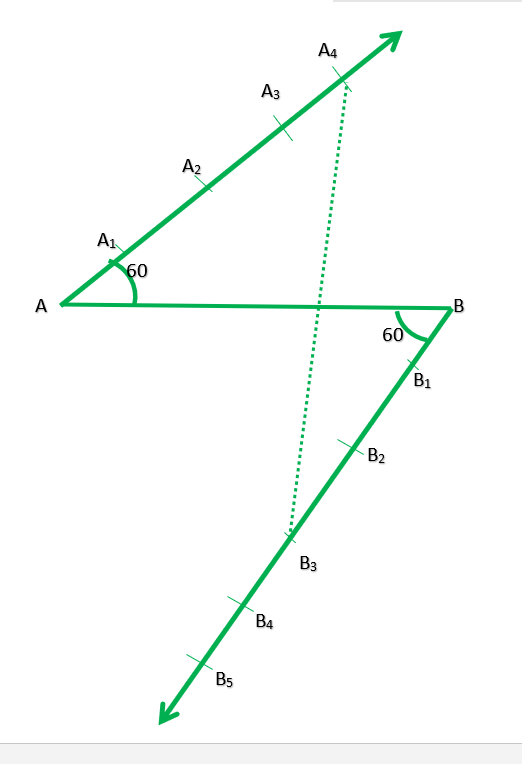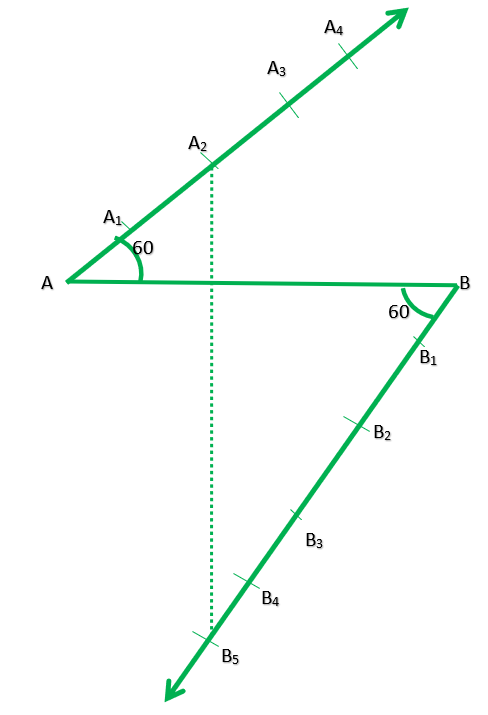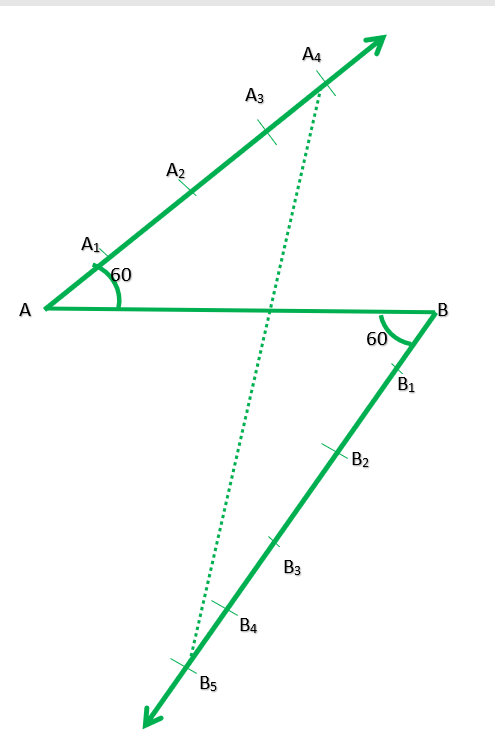# Class 10 RD Sharma Solutions – Chapter 11 Constructions – Exercise 11.1

• Last Updated : 16 May, 2021

### Question 1. Determine a point that divides a line segment of length 12 cm internally in the ratio 2 : 3. Also, justify your construction.

Solution:

Steps of construction:

Hey! Looking for some great resources suitable for young ones? You've come to the right place. Check out our self-paced courses designed for students of grades I-XII

Start with topics like Python, HTML, ML, and learn to make some games and apps all with the help of our expertly designed content! So students worry no more, because GeeksforGeeks School is now here!

Step 1. Draw a line segment AB =12cm.

Step 2. Through the A and B draw two parallel line on each sides of AB.

Step 3. Cut 2 equal parts on AX and 3 equal parts on BY such that AX1=X1X2  and BX1=Y1Y2=Y2Y3

Step 4. Join X2Y3 which intersects AB at PJustification:

In ∆AX2P and ∆BY3P, we have

∠APX2=∠BPY3          {Because they are vertically opposite angle}

∠X2AP=∠Y3BP

∆AX2P and ∆BY3P {Because AA similarity}

Therefore,{Because of C.P.CT}### Question 2. Divide a line segment of length 9 cm internally in the ratio 4 : 3. Also, give justification of the construction.

Solution:

Steps of construction:

Step 1. Draw a line segment AB = 9 cm.

Step 2. Through the points, A and B, draw two parallel lines AX and BY on the opposite side of AB

Step 3. Cut 4 equal parts on AX and 3 equal parts on BY such AX1=X1X2=X2X3=X3X4 and BY1=Y1Y2=Y2Y3

Step 4. Join x4y3 which intersects AB at P.

Therefore,Justification:

In ∆PX4 and ∆BPY3, we have

∠APX4=∠BPY3         {Because they are vertically opposite angles}

∠PAX4=∠PBY3       {Because they are alternate interior angle}

∆APX4     ∆BPY3   {Because AA similarity}

Therefore,{Because of C.P.C.T}### Question 3. Divide a line segment of length 14 cm internally in the ratio 2 : 5. Also, justify your construction.

Solution:

Steps of construction:

Step 1. Draw a line segment AB = 14 cm.

Step 2. Draw a ray AX making an acute angle with AB.

Step 3. From B, draw another ray BY parallel to AX.

Step 4. From AX, cut off 2 equal parts and from B, cut off 5 equal parts.

Step 5. Join 2 and 5 which intersects AB at P.

P is the required point which divides AB in the ratio of 2 : 5 internally.

Justification:

In ∆PX2 and ∆BPY5, we have

∠APX2=∠BPY5         (vertically opposite angles)

∠PAX2=∠PBY5        (Because they are alternate interior angle)

∆APX    ∆BPY5     (Because AA similarity)

Therefore,(Because of C.P.C.T)### Question 4. Draw a line segment of length 8 cm and divide it internally in the ratio 4 : 5.

Solution:

Steps of construction:

Step 1. Draw a line of 8cm.

Step 2. Through the points, A and B, draw two parallel lines AX and BY on the opposite side of AB

Step 3. Cut 4 equal parts AX and 3 equal parts on BY.

Step 4. Join x4y5 which intersects AB at P.

Justification:

In ∆PX4 and ∆BPY5, we have

∠APX4=∠BPY5         (vertically opposite angles)

∠PAX4=∠PBY5       (alternate interior angle)

∆APX4     ∆BPY5   (AA similarity)

Therefore,(Because of C.P.C.T)My Personal Notes arrow_drop_up